# Precalculus : Derivatives

## Example Questions

1 2 6 7 8 9 10 11 12 14 Next →

### Example Question #20 : Determine Points Of Inflection

Find the point(s) of inflection of the following function: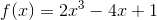Possible Answers: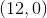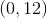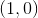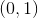Correct answer:Explanation:

To solve, simply differentiate twice, find when the function is equal to 0, and then plug into the first equation.

For this particular function, use to power rule to differentiate.

The power rule states,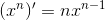.

Also recall that the derivative of a constant is zero.

Applying the power rule and rule of a constant once to the function we can find the first derivative.

Thus,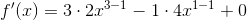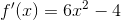From here apply the power rule and rule of a constant once more to find the second derivative of the function.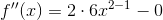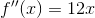Now to solve for the inflection point, set the second derivative equal to zero.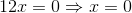From here plug this x value into the original function to find the y value of the inflection point.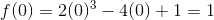Thus, our point is (0,1).

### Example Question #21 : Determine Points Of Inflection

Find the points of inflection for the following function: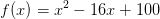Possible Answers: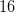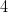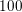Correct answer:Explanation:

To solve, find when the 2nd derivative is equal to 0 and plug it into the first equation. We must use the power rule, as outlined below, to find each derivative.

Power rule:Thus,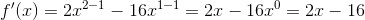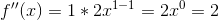Since 2 is never equal to 0, there are no points of inflection.

### Example Question #22 : Determine Points Of Inflection

Determine thevalues for the points of inflection of the following function: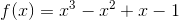Possible Answers: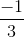Correct answer:Explanation:

To solve, you must set the second derivative equal to 0 and solve for x. To differentiate twice, use the power rule as outlined below:

Power Rule:Therefore: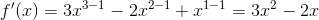Remember, the derivative a constant is 0.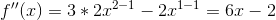Now, set it equal to 0. Thus,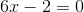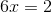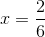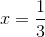1 2 6 7 8 9 10 11 12 14 Next →

### All Precalculus Resources